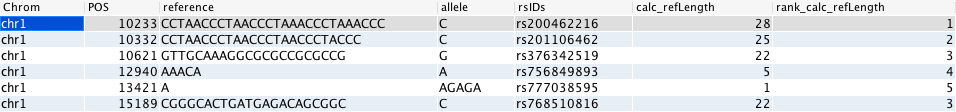Used in: gor/nor

# RANK¶

The RANK command returns the rank of a number within a set of numbers. It compares the value of each cell in the specified column to all the values in the result set and returns the rank of that row in a column labelled “rank_<column_name>”.

The RANK command has two necessary parameters, namely the binsize, which can be set to “chrom”, “genome” or to a numeric value. The column specified in the RANK command must be a numeric column.

Using RANK in a nor query will give an error if `binsize` input parameter is used.

## Usage¶

```gor ... | RANK binsize column [ attributes ]
```
```nor ... | RANK column [ attributes ]
```

## Options¶

 `-o asc | desc` Rank order. By default it is descending. `-q` Report rank distribution, lower rank and equal. `-z` Report z-value = (x-mean)/std. `-c` Report the total count for the bin. `-b` Report the value where the rank is 1. `-rmax number` Report only rows where rank <= number. `-gc cols` Grouping columns (other than bin).

## Examples¶

The example below takes some entries from the `#dbsnp#` table with indels of some length, calculates the length of the reference column in the row and then ranks the value in the column.

```gor #dbsnp# | WHERE len(reference) > 4 OR len(allele) > 4
| CALC refLength len(reference) | PREFIX refLength calc | TOP 6
| RANK genome calc_refLength
```The following query will perform a parallelised gor query that calculates the length of each gene and then ranks them by length and returns the longest gene on each chromosome.

```pgor #genes#
| calc length (gene_end - gene_start)
| rank chrom length -o desc
| where rank_length = 1
```

The next query will return the shortest gene:

```pgor #genes#
| calc length (gene_end - gene_start)
| rank chrom length -o asc
| where rank_length = 1
```